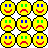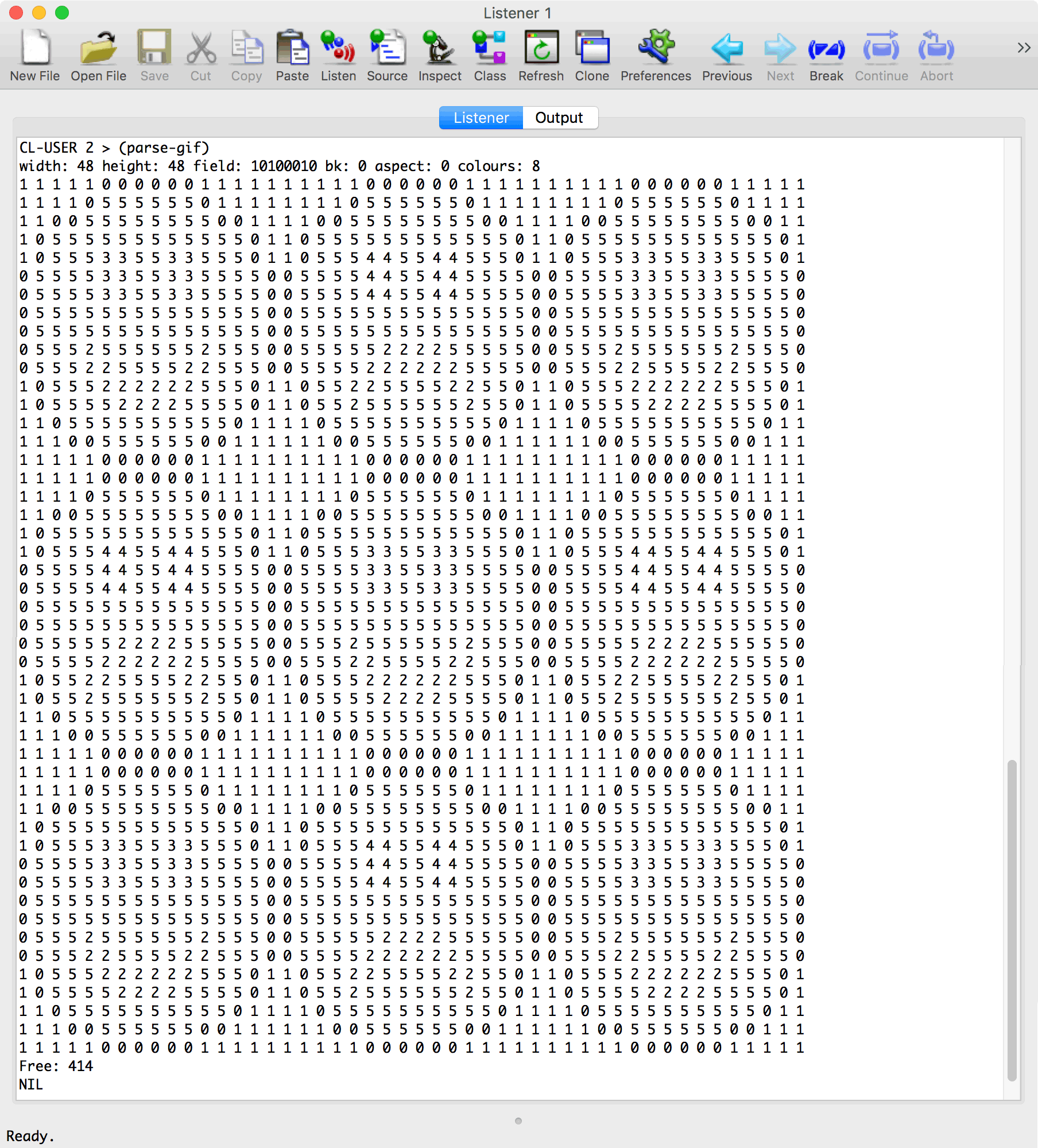## A minimal GIF decoder

16th December 2022

I recently published a small GIF decoder designed to run on AVR microcontrollers .

When I'm writing a tricky algorithm in C I often write it in Lisp first, because it's far easier to get it right, and to debug if it doesn't work. So here is the Lisp prototype of my GIF decoder, in case its useful.

### LZW compression

The GIF format treats the image as a linear stream of pixel colour values. The LZW compression it uses works by finding a sequence of pixels it has already encountered, and it encodes each sequence as a variable-length code of from 3 to 12 bits. These codes are then output as a continuous stream of bits.

Decoding a GIF requires the decoder to divide the input stream into codes containing the correct number of bits. These codes are then looked up in a 4096-element table, to translate them into the appropriate sequence of one or more pixels represented by that code.

It would be very memory intensive if we had to store the complete pixel sequence corresponding to every code in the table. Fortunately, every sequence is equivalent to an earlier sequence, extended by the addition of one pixel. We can therefore represent the sequence as a pointer to the earlier sequence in the table, plus the additional pixel.

The clever thing about LZW compression is that the lookup table doesn't need to be included with the GIF image, because it can be reconstructed dynamically as the code values are read in from the input stream. For a full description of how the GIF format works see the Wikipedia page . I also got a lot of help from articles by Joshua Davies   and Larry Bank .

### Colour table

A GIF image can contain a colour table of up to 256 colours, so there also needs to be a 256-byte array to store this colour table. Each colour is defined by three bytes, giving the colour's R, G, and B components. Since this GIF decoder is intended for displaying images on TFT displays with a 5-6-5 colour space, we can reduce each entry in the colour table to 16 bits.

### Restrictions

This decoder doesn't support: the older GIF87a format, animated GIFs, local colour tables, transparency, interlaced GIFs, or other extensions.

### The compression table

Each element in the compression table used by the GIF decoder is defined by a two-dimensional array, table:

`(let ((table (make-array '(4096 2))))`

In each table entry (aref table n 0) is a pointer to an earlier entry, and (aref table n 1) is the pixel colour of the last pixel in the sequence encoded by this table entry.

A second array colour-table stores the GIF colour table.

The problem of reading variable-length codes from the input file is handled by the routine get-n-bits:

```(defun get-n-bits (n str)
(loop
(unless (< *nbuf* n) (return))
;; Enough left in block?
(when (zerop *block*)
(setq *buf* (logior (ash (read-byte str) *nbuf*) *buf*))
(decf *block*)
(incf *nbuf* 8))
(let ((result (logand *buf* (1- (ash 1 n)))))
(setq *buf* (ash *buf* (- n)))
(decf *nbuf* n)
result))```

A call to (get-n-bits n) returns the next n-bit code from the file. This maintains a bit buffer in the global variable *buf*, and *nbuf* specifies how many significant bits are still available in *buf*. If there are fewer than n bits in the buffer, read-byte is called to read in another 8 bits and append them to the end of the buffer.

A further complication is that the bit stream is divided into blocks of up to 255 bytes, with each block preceded by a length byte. The global variable *block* stores the number of bytes remaining in the current block. If this reaches zero, it is reset by reading in another length byte.

### Getting the first character of a sequence

Given a compression table element c, first-pixel follows the pointers to find the first pixel colour in the sequence it represents:

```(defun first-pixel (table c)
(let ((rest (aref table c 0))
(this (aref table c 1)))
(cond
((= -1 rest) this)
(t (first-pixel table rest)))))```

### Outputting a sequence

The routine plot-sequence plots the sequence of pixels represented by the compression table element c.

```(defun plot-sequence (table c)
(let ((rest (aref table c 0))
(this (aref table c 1)))
(unless (= -1 rest) (plot-sequence table rest))
(plot this)))```

This effectively finds the first pixel in the sequence, by following the pointers back until a -1 pointer is reached, then finds the next pixel in the sequence, and so on, ending with the last pixel:

`(aref table c 1)`

### Displaying the output

For debugging purposes this version of the GIF decoder prints the output as a series of digits to the Listener:

```(defun plot (c)
(format t "~a " c)
(incf *x*)
(when (= *x* *width*) (setq *x* 0) (incf *y*) (format t "~%")))```

It could easily be modified to plot points in the appropriate colours from colour-table.

### Skipping bytes

The routine skip-n-bytes is called to skip a series of unneeded bytes in the input file:

```(defun skip-n-bytes (n str)

### Power of two test

The routine power-2-p checks that its argument is a power of two. It is used to increase the bit length of codes being read when the size of the compression table reaches a power of two:

```(defun power-2-p (x)
(zerop (logand x (1- x))))```

### Displaying the GIF image

Finally, here's the definition of parse-gif:

```(defun parse-gif ()
(let ((file (capi:prompt-for-file "GIF File" :pathname *last-file*)))
(setq *last-file* file)
(with-open-file (str file :element-type '(unsigned-byte 8))
(skip-n-bytes 6 str) ; id and version
(colbits (max (1+ (logand fld 7)) 2))
(colours (expt 2 colbits))
(colour-table (make-array colours))
(clr colours)
(end (1+ colours))
(free (1+ end))
(bits (1+ colbits)))
(format t "width: ~a height: ~a fld: ~b bk: ~a aspect: ~a colours: ~a~%"
width height fld bk aspect colours)
(setq *width* width)
;; Parse colour table
(dotimes (colour colours)
(setf (aref colour-table colour)
;; Make and initialise the compression table
(let ((table (make-array '(4096 2))))
(dotimes (n colours) (setf (aref table n 0) -1 (aref table n 1) n))
;; Parse blocks
(loop
(cond
;; Parse image descriptor
(skip-n-bytes 10 str)
(setq *nbuf* 0)
(setq *buf* 0)
(setq *block* 0)
(setq *x* 0)
(let (code last)
(loop
(setq last code)
(setq code (get-n-bits bits str))
(cond
((= code clr)
(setq free (1+ end))
(setq bits (1+ colbits))
(setq code nil))
((= code end) (return))
((null last)
(plot-sequence table code))
((< code free) ; Found in table
(setf (aref table free 0) last
(aref table free 1) (first-pixel table code))
(plot-sequence table code)
(incf free)
(when (power-2-p free) (incf bits)))
(setf (aref table free 0) last
(aref table free 1) (first-pixel table last))
(plot-sequence table free)
(incf free)
(when (power-2-p free) (incf bits)))
(t nil)))
(skip-n-bytes 1 str)
(format t "Free: ~a~%" free)))
;; Skip extension block
(skip-n-bytes (1+ length) str)))
(return))
(t nil)))))))))```

The routine parse-gif first reads in the GIF header, which specifies the width and height of the image, and the global colour table. If the image has c colours it then initialises the first c entries in the compression table, table, with the corresponding colour index, c. A further two entries are reserved for two special codes: clear, which resets the compression table if it is full, and end which indicates the end of the image block. The first free entry in the table is therefore at c+2.

Next parse-gif checks for three types of block: an image block, prefixed by #x2C, an extension block prefixed by #x21 which is ignored, and a terminating byte #x3B that ends the file.

In the image block the most important variables are free, that specifies the index of the next free element in the compression table, and bits, that specifies the current size of the variable-length codes.

The compression table is extended as follows, based on each code that is read in from the input file:

• If code < free the code is already in the table. A new entry is added to the table consisting of a pointer to the last sequence, followed by the first pixel in the current sequence.
• If code = free the code is not already in the table. A new entry is added to the table consisting of a pointer to the last sequence, followed by the first pixel in the last sequence.

In each case the sequence corresponding to code is output.

This continues until either a clear code is encountered, in which case the compression table is cleared and processing continues, or an end code is encountered, in which case the image is finished.

### Running the program

I tested the program with a 48 x 48 pixel 8-colour GIF:Here's the program output, with each colour printed as a different digit in the Listener:Here's the complete program: Minimal GIF decoder program.

1. ^ Minimal GIF Decoder on Technoblogy.
2. ^ GIF on Wikipedia.
3. ^ How LZW (GIF) Compression Works on Command Line Fanatic.
4. ^ Inside the GIF File Format on Command Line Fanatic.
5. ^ A Low Memory GIF Decoder on Follow me down the optimization rabbit hole.